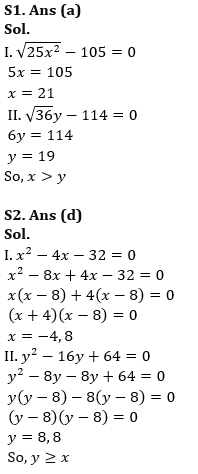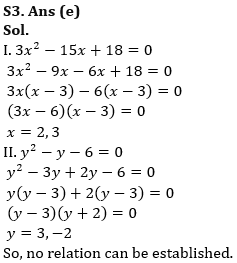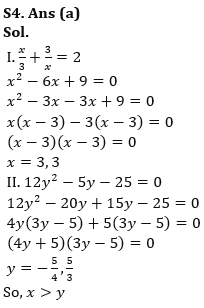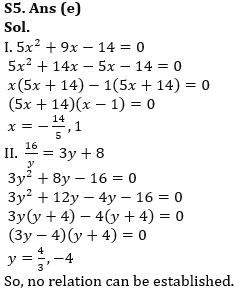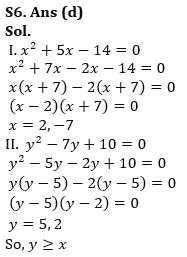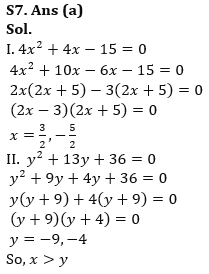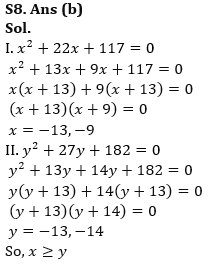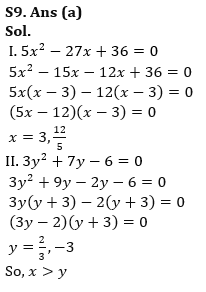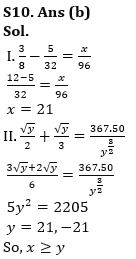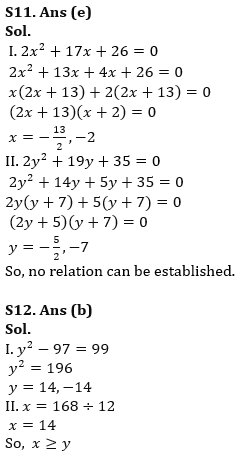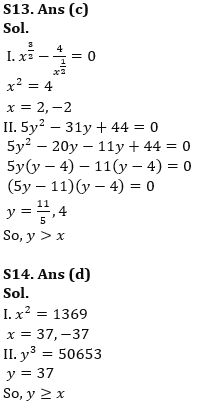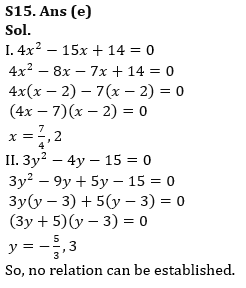Latest Banking jobs   »   Quantitative Aptitude Quiz For RBI Grade...

# Quantitative Aptitude Quiz For RBI Grade B/ ECGC PO/ SIDBI Grade A Prelims 2022- 4th May

Directions (1-15): In each of these questions, two equation (I) and (II) are given. You have to solve both the equations and give answer.

(a) If x>y
(b) If x≥y
(c) If x<y
(d) If x≤y
(e) If x = y or no relation can be established between x and y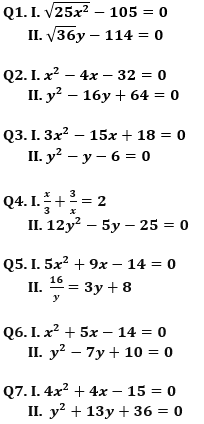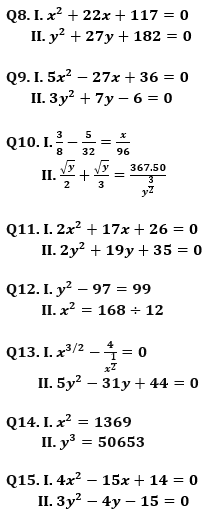Solutions